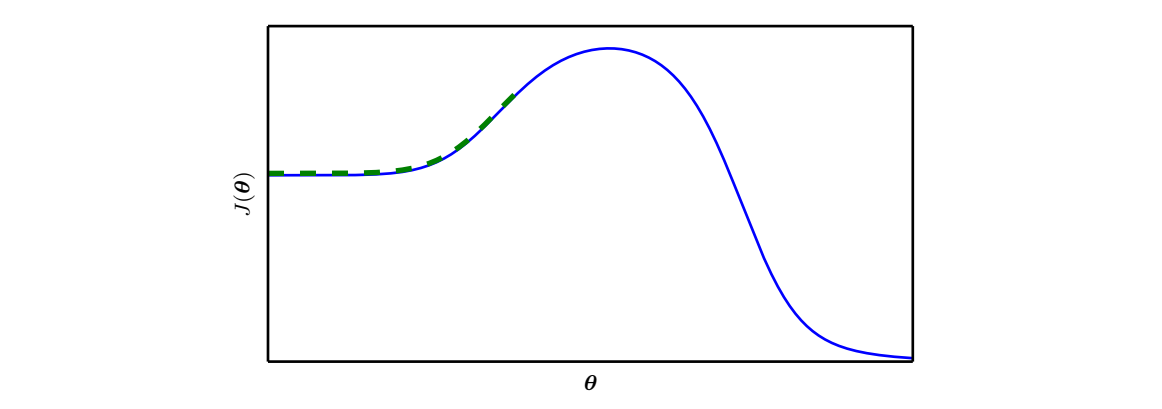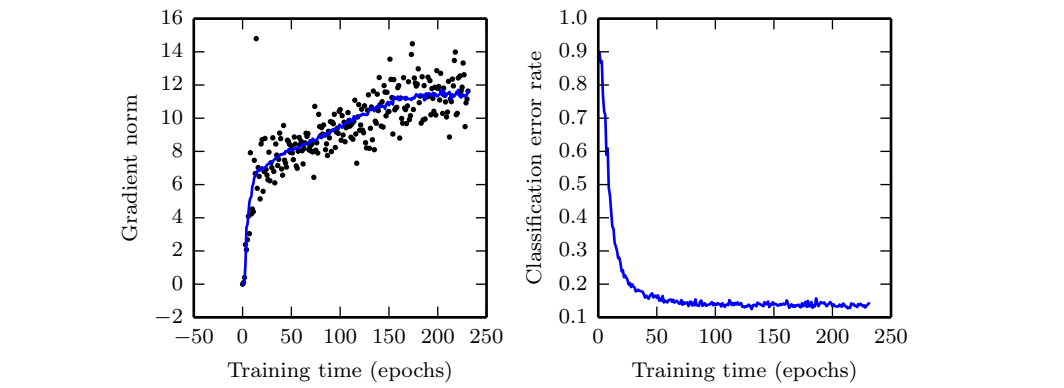• 局部收敛和全局收敛
千次阅读
2010-12-26 20:26:15
通常考察一个算法的性能通常用局部搜索能力和全局收敛能力这两个指标。局部搜索是指能够无穷接近最优解的能力，而全局收敛能力是指找到全局最优解所在大致位置的能力。

人同样不也有与这类似两种能力么？局部搜索能力对应人对于自己所遇到的事情刨根究底，从而精通的能力。举个例子，这种能力可以是与人交往的能力，学好功课的能力，创业的能力，做饭的能力，甚至蹭饭的能力。。。而全局搜索能力则对应着一个人的是否对全局信息有较好的把握，在不断失败的尝试中，然后找到了自己喜欢的一种生活状态。

局部搜索能力和全局搜索能力，缺一不可。如果一味强调精而忽视广，就会陷入极其狭隘的空间，成为了井底之蛙。如果过于喜新厌旧，浅尝辄止，则会失去了体验生活独到之处的机会。

我们不也正是这样么？高中毕业时，大家走向了不同的大学，从此我们的搜索空间开始不同。有的人到了大学，在“娱乐”这个解附近徘徊不前，精通“娱乐”这门艺术；有的人喜欢上了“学习”，学习使他们产生了一种偏执，“万般皆下品，惟有读书高”；更多的人游荡在大学中我们可能接触到的解附近，寻寻觅觅想找到自己梦中的归宿，苦苦追寻想看清自己的未来。

向最优解的导向，对于任何智能算法的性能都是很重要的。同样，我相信，对“人生最优解”的渴望，将引导着我走向自己人生最美的地方。算法
更多相关内容
• 大部分全局收敛理论处理的是保证全局收敛的环境与条件，其中一个重要的理论如下： 定 理 1 ： \textbf{定理1：} 令A表示X上的算法并假设初始点 x 0 \textbf{x}_0 将产生一个无穷序列 { x k } ∞ k = 0 \{\textbf{...

$\textbf{平时我看起来很坚强，}$
$\textbf{可是遇到你，我会变成另一个模样。}$
$\textbf{我不想考虑太多，}$
$\textbf{因为追随内心，你已经走进我的心墙。}$
$\textbf{我想让你知道，}$
$\textbf{我从未想过放手，}$
$\textbf{只愿陪你到永远。}$
—— $\qquad\qquad\quad\textbf{——畅宝宝的傻逼哥哥}$

如果一个算法满足这样的性质：任意的初始点 x0X $\textbf{x}_0\in X$都会产生一个收敛的点序列 {xk}k=0 $\{\textbf{x}_k\}_{k=0}^\infty$，那么称该算法是全局收敛的。实际上，如果某些条件不满足，甚至非常有效的算法都会失效。例如算法可能产生不收敛的序列或者收敛的点不是所求的解，存在一些导致算法失败的因素，但是如果我们很清楚的了解他们，那么我们就能采取某些避免失败的措施，所以全局收敛成为理论学者与实践者共同的兴趣。

大部分全局收敛理论处理的是保证全局收敛的环境与条件，其中一个重要的理论如下：

1 $\textbf{定理1：}$令A表示X上的算法并假设初始点 x0 $\textbf{x}_0$将产生一个无穷序列 {xk}k=0 $\{\textbf{x}_k\}_{k=0}^{\infty}$，其中

xk+1A(xk)

如果该算法的解集S与下降函数 D(xk) $D(\textbf{x}_k)$存在，使得

• 所有点 xk $\textbf{x}_k$包含在X的紧子集中
• D(xk) $D(\textbf{x}_k)$满足下降函数的定义且
• A的映射对S外的所有点都封闭

那么任何收敛序列 {xk}k=0 $\{\textbf{x}_k\}_{k=0}^{\infty}$的极限都是解。

$\textbf{证明：}$这个定理的证明分两部分。对a部分，我们假设 x̂  $\hat{\textbf{x}}$是任何序列 {xk}k=0 $\{\textbf{x}_k\}_{k=0}^{\infty}$子序列 {xk}kI $\{\textbf{x}_k\}_{k\in I}$的极限，其中I是整数的一个集合，并且说明 D(xk) $D(\textbf{x}_k)$对无限序列 {xk}k=0 $\{\textbf{x}_k\}_{k=0}^{\infty}$收敛。对b部分，我们说明 x̂  $\hat{\textbf{x}}$是集合S的解。

证明的第二部分非常依赖于魏尔斯特拉斯定理，如果W是紧集，那么序列 {xk}k=0 $\{\textbf{x}_k\}_{k=0}^{\infty}$的极限点在W中，其中 xkW $\textbf{x}_k\in W$。如果集合W是闭的，那么它也就紧集。如果W边界上的所有点都属于W，那么W是闭集。如果W能被一个有限半径的超球包围，那么W是有界集。魏尔斯特拉斯定理的一个结论是 {xk}k=0 $\{\textbf{x}_k\}_{k=0}^{\infty}$的任何子序列 {xk}kI $\{\textbf{x}_k\}_{k\in I}$的极限位于集合 W¯={xk:kI} $\bar{W}=\{\textbf{x}_k:k\in I\}$中，因为 W¯ $\bar{W}$是W的子集，所以它也是紧集。

(a)因为 D(xk) $D(\textbf{x}_k)$在X上是连续的且 x̂  $\hat{\textbf{x}}$是序列 {xk}kI $\{\textbf{x}_k\}_{k\in I}$的极限，所以存在正数与整数K使得当 kK $k\geq K$

D(xk)D(x̂ )<ε

其中 kI $k\in I$，因此 D(xk) $D(\textbf{x}_k)$对子序列 {xk}kI $\{\textbf{x}_k\}_{k\in I}$收敛。然而我们还必须证明 D(xk) $D(\textbf{x}_k)$对无限序列 {xk}k=0 $\{\textbf{x}_k\}_{k=0}^{\infty}$收敛。

对于任意 kK $k\geq K$，我们有

D(xk)D(x̂ )=[D(xk)D(xK)]+[D(xK)D(x̂ )]

如果 k=K $k=K$，那么

D(xK)D(x̂ )<ε

如果 kK $k\geq K$，那么 D(xk)D(xK) $D(\textbf{x}_k)\leq D(\textbf{x}_K)$，因此

D(xk)D(xK)0

由此可得对任意 kK $k\geq K$

D(xk)D(x̂ )<ε

因此

limkD(xk)=D(x̂ )

即当 xkx̂  $\textbf{x}_k\to\hat{\textbf{x}}$ D(xk) $D(\textbf{x}_k)$相对于无限序列收敛。

(b)假设 x̂  $\hat{\textbf{x}}$不在解集中，因为子序列 {xk+1}kI $\{\textbf{x}_{k+1}\}_{k\in I}$的元素属于属于紧集，根据魏尔斯特拉斯定理可知存在紧子集 {xk+1:kI¯I} $\{\textbf{x}_{k+1}:k\in\bar{I}\subset I\}$使得 xk+1 $\textbf{x}_{k+1}$收敛到极限 x¯ $\bar{\textbf{x}}$。根据部分(a)，我们说明了

limkD(xk+1)=D(x¯)

因此

D(x¯)=D(x̂ )

另一方面

xkx̂ xk+1x¯for kI¯for xk+1A(x)

根据假设 x̂ S $\hat{\textbf{x}}\notin S$，并且A对S外的点是闭的，我们有

x¯A(x̂ )

因此

D(x¯)<D(x̂ )

得出矛盾，因为 {xk}k=0 $\{\textbf{x}_k\}_{k=0}^{\infty}$的任何收敛子序列的极限是解。

对于简单的情况，上面的定理说明，如果

• 算法生成的点在有限的 En $E^n$空间中
• 可以找到满足要求的下降函数
• 算法在解邻域的外边是封闭的

那么算法是全局收敛的。进一步，我们可以在有限次迭代下得到近似解，因为 {xk}k=0 $\{\textbf{x}_k\}_{k=0}^{\infty}$的任何有限子序列的极限都是解。

定理1的推论同样非常重要：

1 $\textbf{推论1：}$如果定理1的条件成立，解集S由一个点 x̂  $\hat{\textbf{x}}$组成，那么序列 {xk}k=0 $\{\textbf{x}_k\}_{k=0}^{\infty}$收敛到 x̂  $\hat{\textbf{x}}$

$\textbf{证明：}$如果我们假设有一个子序列 {xk}kI $\{\textbf{x}_k\}_{k\in I}$不收敛到 x̂  $\hat{\textbf{x}}$，那么对于所有的 kI,ε>0 $k\in I,\varepsilon>0$

xkx̂ >ε

集合 {xkII} $\{\textbf{x}_k\in I^{'}\subset I\}$是紧集， {xk} $\{\textbf{x}_k\}$

展开全文• 给出了保证算法全局收敛的充分条件，即全局性假设条件单调性假设条件，进而依据提出的全局收敛的充分条件，设计了具有柯西随机高斯随机性质的粒子群算法。实验结果表明，本文提出的具有全局收敛性的粒子群算法...
• 针对这些缺陷, 在改进Heaviside函数的基础上, 引入全局高斯拟合项, 并且对局部高斯拟合项和全局高斯拟合项的权重系数均采用自适应的方法进行调整, 得到基于局部和全局高斯拟合的主动轮廓分割模型。改进模型不仅能...
• 牛顿迭代法的收敛性分为局部收敛性与全局收敛性。 **局部收敛性：**若α是f(x)=0的一个单根, f(α)=0,f’(α)≠0,ϕ’(α)=0, ϕ’’(α)=f’’(α)/f’(α), 则在根α附近Newton 法是局部收敛的, 并且是二阶收敛的...

# 牛顿迭代法收敛性的一点讨论

今天在使用牛顿迭代法求方程的数值解时，发现其一会儿收敛，一会儿不收敛，于是认证研究了下牛顿迭代法的收敛条件。
牛顿迭代法的收敛性分为局部收敛性与全局收敛性。
**局部收敛性：**若α是f(x)=0的一个单根, f(α)=0,f’(α)≠0,ϕ’(α)=0, ϕ’’(α)=f’’(α)/f’(α), 则在根α附近Newton
法是局部收敛的, 并且是二阶收敛的。这个附近指的是充分接近。要多接近呢？似乎没有进一步的证明。
这就决定了牛顿迭代法的初值选取非常重要，只有在解的附近选初值才具有局部收敛性。可是证明去找解的附近呢？一种方法是先用二分法找一个大概的解，再用牛顿法求解。
然而，对于某些函数，初值离解很远也能收敛，这就要谈到全局收敛性了。
全局收敛性：
设f(x)在有根区间[a, b]上二阶导数存在，且满足
(1) f(a)f(b)<0;
(2) f’(x)≠0, x∈[a, b];
(3) f’’(x)不变号, x∈[a, b];
(4)初值x0 ∈[a, b]且使f’’(x0) *f(x0)>0;
则Newton迭代法收敛于f(x)=0在[a, b]内的惟一根。
其中，条件（2）和（3）要求函数在区间内为凸或凹函数，条件（4）又规定了初值接近解的方向，算是比较苛刻的条件。以f(x)=lnx为例，若选择初值x0为4，则第一次迭代后的值x1为-1.505，超过了定义域，就不收敛了。可如果初值选择0.5，则是收敛的。展开全文算法
• 针对标准粒子群算法(PSO)全局局部搜索能力相互制约的缺点,提出一种带有独立局部搜索机制、多区域搜索策略渐近收敛能力的新型PSO算法(ILS-PSO).设计新的简化参数的全局搜索公式、非劣解邻域局部搜索公式当前最...
• 面积项通过引入一个全局指示函数，加快了模型的收敛速度，且能避免陷入局部极小。惩罚项约束水平集函数逼近符号距离函数，使模型无需重新初始化，减少了分割时间。此外，为了实现对图像中感兴趣区域的分
• b) 它收敛全局最优，即使该函数包含许多局部函数。 该算法适用于维数相对较低的函数：最多约 10-20 个变量。 如果维度太高，函数可能无法定位全局最小值。 一个有用的测试是运行该函数几次，检查它是否找到了...matlab
• 针对局部全局一致性学习(LLGC) 算法的分类精度在很大程度上取决于控制参数的合理设置问题, 提出一 种少参数的简洁局部全局一致性学习(BB-LLGC). 简化图上的目标函数, 使其不受参数alpha的影响. 另外, 在标签传递...
• 利用粒子群算法强大的全局优化能力，并行计算的快速收敛性，对BP神经网络进行初始权值、阈值寻优，再使用LM算法的快速局部寻优能力，避免了BP算法精度波动大，易陷入局部极小值的缺陷
• 为了研究蝙蝠算法的收敛性，本文基于随机搜索算法的全局收敛性判断准则对蝙蝠算法的收敛性...结果表明，蝙蝠算法不完全满足随机搜索优化算法的2个全局收敛准则，无法确保全局收敛，因此蝙蝠算法属于局部搜索优化算法．
• 如果该方向在局部改进很大，但并没有指向代价低得多的遥远区域，那么我们有可能在单点处克服以上所有困难，但仍然表现不佳。Goodfellow等人认为大部分训练的运行时间取决于到达解决方案的轨迹长度。如下图所示，学习...

分类目录：《机器学习中的数学》总目录
相关文章：
· 病态
· 局部极小值
· 高原、鞍点和其他平坦区域
· 梯度消失和梯度爆炸
· 非精确梯度
· 局部和全局结构间的弱对应

迄今为止，我们讨论的许多问题都是关于损失函数在单个点的性质——若 J ( θ ) J(\theta) 是当前点 θ \theta 的病态条件，或者 θ \theta 在悬崖中，或者 θ \theta 是一个下降方向不明显的鞍点，那么会很难更新当前步。

如果该方向在局部改进很大，但并没有指向代价低得多的遥远区域，那么我们有可能在单点处克服以上所有困难，但仍然表现不佳。Goodfellow等人认为大部分训练的运行时间取决于到达解决方案的轨迹长度。如下图所示，学习轨迹将花费大量的时间探寻一个围绕山形结构的宽弧。大多数优化研究的难点集中于训练是否找到了全局最小点、局部极小点或是鞍点，但在实践中神经网络不会到达任何一种临界点。下图表明神经网络通常不会到达梯度很小的区域。甚至，这些临界点不一定存在。例如，损失函数 − log ⁡ p ( y ∣ x ; θ ) -\log p(y|x;\theta) 可以没有全局最小点，而是当随着训练模型逐渐稳定后，渐近地收敛于某个值。对于具有离散的 y y 和Softmax分布 p ( y ∣ x ) p(y|x) 的分类器而言，若模型能够正确分类训练集上的每个样本，则负对数似然可以无限趋近但不会等于零。同样地，实值模型 p ( y ∣ x ) = N ( y ; f ( θ , β − 1 ) ) p(y|x)=N(y;f(\theta,\beta^{-1})) 的负对数似然会趋向于负无穷——如果 f ( θ ) f(\theta) 能够正确预测所有训练集中的目标 y y ，学习算法会无限制地增加 β \beta 。上图给出了一个失败的例子，即使没有局部极小值和鞍点，该例还是不能从局部优化中找到一个良好的代价函数值。未来的研究需要进一步探索影响学习轨迹长度和更好地表征训练过程的结果。许多现有研究方法在求解具有困难全局结构的问题时，旨在寻求良好的初始点，而不是开发非局部范围更新的算法。

梯度下降和基本上所有的可以有效训练神经网络的学习算法，都是基于局部较小更新。之前的小节主要集中于为何这些局部范围更新的正确方向难以计算。我们也许能计算目标函数的一些性质，如近似的有偏梯度或正确方向估计的方差。在这些情况下，难以确定局部下降能否定义通向有效解的足够短的路径，但我们并不能真的遵循局部下降的路径。目标函数可能有诸如病态条件或不连续梯度的问题，使得梯度为目标函数提供较好近似的区间非常小。在这些情况下，步长为 ϵ \epsilon 的局部下降可能定义了到达解的合理的短路经，但是我们只能计算步长为 δ < < ϵ \delta<<\epsilon 的局部下降方向。在这些情况下，局部下降或许能定义通向解的路径，但是该路径包含很多次更新，因此遵循该路径会带来很高的计算代价。有时，比如说当目标函数有一个宽而平的区域，或是我们试图寻求精确的临界点（通常来说后一种情况只发生于显式求解临界点的方法，如牛顿法）时，局部信息不能为我们提供任何指导。在这些情况下，局部下降完全无法定义通向解的路径。在其他情况下，局部移动可能太过贪心，朝着下坡方向移动，却和所有可行解南辕北辙，或者是用舍近求远的方法来求解问题。

不管哪个问题最重要，如果存在一个区域，我们遵循局部下降便能合理地直接到达某个解，并且我们能够在该良好区域上初始化学习，那么这些问题都可以避免。最终的观点还是建议在传统优化算法上研究怎样选择更佳的初始化点，以此来实现目标更切实可行。

一些理论结果表明，我们为神经网络设计的任何优化算法都有性能限制。通常这些结果不影响神经网络在实践中的应用。

一些理论结果仅适用于神经网络的单元输出离散值的情况。然而，大多数神经网络单元输出光滑的连续值，使得局部搜索求解优化可行。一些理论结果表明，存在某类问题是不可解的，但很难判断一个特定问题是否属于该类。其他结果表明，寻找给定规模的网络的一个可行解是很困难的，但在实际情况中，我们通过设置更多参数，使用更大的网络，能轻松找到可接受的解。此外，在神经网络训练中，我们通常不关注某个函数的精确极小点，而只关注将其值下降到足够小以获得一个良好的泛化误差。对优化算法是否能完成此目标进行理论分析是非常困难的。因此，研究优化算法更现实的性能上界仍然是学术界的一个重要目标。

展开全文人工智能 机器学习 深度学习 优化
• 提出一种全局竞争声搜索(GCHS) 算法, 给出随机局部平均和全局平均声的概念, 建立竞争搜索机制, 实现每次迭代产生两个声向量并进行竞争选择. 设计自适应全局调整和局部学习策略, 平衡算法的局部搜索和全局...
• 利用遗传算法早熟的特点, 构造出一种快速收敛的混合算法来求解优化问题, 并...能力,并采用 Nelder- Mead 单纯形法来加强算法的局部搜索能力,加快了算法的收敛速率。模拟实验表 明,该方法具有高效性鲁棒性。</p>
• 本书第 9 章的插图。 我们涵盖遗传算法以及基于牛顿的优化器。... 特别是，我们提供了一种 SQP 方法，它是一种全局收敛局部优化器。 我们可以指定范围广泛的边界条件。 所有这些都应用于金融定价模型的校准matlab
• 内容：常见的局部优化和全局优化算法。 时间：2019.01.05 梯度下降，牛顿法，共轭梯度法（Python + Matlab），找到空间表面的极值点（3D） 温馨提示： 梯度下降算法速度慢，迭代次数大，最终结果是近似的。 牛顿法...
• 前段时间在看深度学习的内容，对于全局最小值和局部最小值这两个概念之前理解的不太深入，总是容易混淆，看了西瓜书之后明白了，特此总结一下，加深印象。 1. 概念 局部最小值是在某一区域内，函数的取值达到了...机器学习 深度学习
• 为了解决布谷鸟搜索算法后期收敛速度慢、求解精度不高、易陷入局部最优等缺陷，提出了一种基于Powell局部搜索策略的全局优化布谷鸟搜索算法。算法将布谷鸟全局搜索能力与Powell方法的局部寻优性能有机地结合，并根据...布谷鸟搜索算法 莱维飞行
• 针对可缩放区域拟合(RSF)模型对初始轮廓敏感的缺点，提出了一种结合全局和局部图像信息的变分水平集活动轮廓模型。该模型设计了一个灰度域上的核函数，将其与RSF模型空域核的线性组合作为局部能量项，弥补了采样权值...
• 对于方程组 F(x)=0,其中 F: Rn→Rn是局部 Lipschitz连续但不可微的,提出了光滑 BFGS方法,即利 用光滑函数逼近非光滑函数,每一步用 BFGS公式计算修正矩阵,并采用与...x是 Lipschitz连续的条件下,证明了算法的全局收敛性.
• 考虑复合非光滑最优化问题minh(f(x))，其中，是一个局部Hpselfitzian函数，h是一个连续可微凸函数.本文给出了复合非光滑最优化问题的一个线搜索算法，并且在一定条件下证明了该算法的全局收敛性.
• 针对狼群算法求解复杂函数时容易陷入局部极值、计算耗费大、学习能力差等局限性, 提出一种狼群智能算法. 首先, 通过构建智能猎杀行为提高... 实验结果表明, 所提出算法具有全局收敛性强、计算耗费低、寻优精度高等优势.
• 文章目录一、理论基础1、鲸鱼算法2、改进后算法（1）非线性收敛因子（2）局部扰动（3）改进算法伪代码二、仿真实验与结果分析三、参考文献四、Matlab仿真程序 一、理论基础 1、鲸鱼算法 请参考这里。 2、改进后算法 ...matlab
• 通过其他声向量之间不断回溯交互的随机学习策略,提高算法局部搜索性能;用由两种不同学习策略随机交叉动态产生的新和声与反向声二者较优的个体更新声记忆库,提高算法的搜索性能.将OLGHS算法与其他启发式优化...
• 为了解决布谷鸟搜索算法后期收敛速度慢、求解精度不高、易陷入局部最优等缺陷，提出了一种基于Powell局部搜索策略的全局优化布谷鸟搜索算法。算法将布谷鸟全局搜索能力与Powell方法的局部寻优性能有机地结合，并根据...
• 但是在多变量的时候，Hessian有多个不同的特征值，这时候各个特征值就可能会有更复杂的分布，如有正有负的不定型有多个退化特征值（零特征值）的半定型 在后两种情况下，是很难找到局部极值的，更别说全局最优了。...神经网络 算法 机器学习 人工智能 深度学习
• 图像特征分为全局特征和局部特征两种，其中全局特征代表了图像的整体表现特性，比如颜色直方图，而局部特征代表了图像的局部特性，往往能够从一幅图片中提取出若干个数量不等的局部特征， 这些局部特征组合起来代表...
• 今天介绍美国华盛顿大学保罗·艾伦计算机科学与工程学院的Su-In Lee团队在nature mechine intelligence 2020的论文，该论文提出了一种基于博弈论沙普利值的TreeExplainer方法，用于从局部全局的树模型解释性研究。...
• 研究了当前在结构优化设计中处理全局约束问题的方法，进而提出了局部刚度修正法，即只要修改部分构件的刚度就可使全局约束条件得到满足。通过算例工程实践表明，对规模为1000个节点、50个设计变量的杆系结构，在...
• 针对微分进化（differential evolution，DE）算法在进化后期收敛速度慢、收敛精度低、易陷入局部最优解等缺点。通过改进DE的变异方程，并引入一种新的控制参数自适应策略，提出了一种改进自适应微分进化（improved ...微分进化...